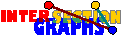# Intersection Number

It seems to be a natural goal to represent graphs as intersection graphs of (simple) hypergraphs with minimum vertex set. The intersection number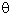(G) of a graph is this minimum number, i.e. the smallest n for which G is the intersection graph of some hypergraph (A,(Sx/xV)) with |xV Sx|=n.[EGP66]: The edge set of every n-vertex graph without isolated vertices can be partitioned into [ n2/4 ] edges or triangles.
 Proof: We use induction on n. Let G have n vertices, none of them isolated. Since [n2/4] = [(n-1)2/4]+[n/2], we only have to show how such a partition for some (n-1)-vertex subgraph GÇ of G (which has to exist, by induction hypothesis) can be extended to some partition for the whole graph. If G has some vertex x of degree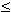[n/2], this is obvious---we choose GÇ = G-x and add all edges incident with x. So assume in the following(G)=[n/2]+r, where r>0, and choose a vertex x where dG(x)=(G). We show that G[N(x)] must have r independent edges e1,...,er. For, otherwise every neighbor y of x not occuring in any of these edges has at most 2r-2 neighbors in G[N(x)], and obviously at most n-dG(x) outside. Thus dG(y)2r-2+n-([n/2]+r) < [n/2]+r, a contradiction. Now obtain GÇ from G by first deleting x and then e1,...,er. To the existing partition of the edge set of GÇ, we add all r triangles formed by x and the ei, and we add all the remaining [n/2]-r edges incident with x.

The result is sharp, as can be seen by the complete bipartite graphs K[n/2],lceil n/2 rceil.

Using dualization, there follows that every graph is the intersection graph of some linear hypergraph with at most [n2/4] vertices. If there are hyperedges of cardinality 1, then this hypergraph is not necessarily simple, but it is also possible to express G as intersection graph of some simple hypergraph with at most [n2/4] vertices.[KSW78]: Finding a minimum covering of the edges of a graph by complete subgraphs is NP-complete.
 Proof: Given a graph G, the minimum cardinality -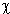(G) of a set of complete subgraphs covering all vertices of G is the chromatic number of the complement of G, and is therefore NP-complete. Let GÇ be constructed from G by adding m+1 new vertices a1,a2,..., am+1, all adjacent to the vertices of G, where m is the number of edges of G. Let S1,S2,...Sk be a minimum covering of the vertices of G by complete subgraphs. Then all k(m+1) complete graphs Si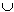{aj}, together with all edges of G cover all edges of GÇ, therefore(GÇ)(m+1)-(G)+m. Now assume we had some polynomial-time algorithm for finding minimum covering of the edges by complete graphs for every graph. Then we find such a cover for GÇ. For every 1im+1, let Mi the set of those members of our cover containing ai. Since the members of each Mi cover all edges from ai to vertices of G, they cover all vertices of G, whence |Mi|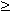-(G) for every 1im+1. We claim min1im+1|Mi| = -(G), a contradiction to the NP-completeness for computing -(G). For, assume |Mi| > -(G) for every 1im+1. Then our optimal cover contains at least (m+1) (-(G)+1) elements, a contradiction to the formula above.

Using essentially the same idea, but adding Ce+1 new vertices instead of e+1, it is possible to show that, for every fixed constant C1, every algorithm that always finds a covering of the edges of every graph G of cardinality at most C(G), could be used to find a coloring of every graph G using at most C(G)+d colors. But such a polynomial algorithm would imply P=NP by [LY93] and could therefore not be expected to exist.

Concerning random graphs G(n,1/2) we get (1-) (n/2 ln n)2(G)O((n/ln n)2(ln ln n)) for almost every graph [BESW93].

In the same way, the p-intersection numberp(G) of a graph G is the smallest vertex set of a hypergraph whose p-intersection graph equals G. Concerning the graphs Kn,n it seems likep ~/p, sincep( Kn/2,n/2) = (n2/4p)(1+o(n)) [EGR96], [F97].

Back to the start page for intersection graphs.

Erich Prisner